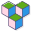CppBuzz# Python program to find LCM of two numbers

This program create a double dimention int arrays, suffle its elements and then find saddle point if any

# Author: CppBuzz.com
# This program calculates the LCM of the two numbers
# Date: 22nd Aug 2020

```
def LCM(number1, number2):
"""This function calculates LCM of two numbers inputed by the user"""
maximum = max(number1, number2)
i = maximum
while True:
if i % number1 == 0 and i % number2 == 0:
lcm = i
break
i += maximum

return lcm

if __name__ == '__main__':
userInput1 = int(input('Enter first number: '))
userInput2 = int(input('Enter second number: '))
print('LCM of {} and {} is {}'.format(userInput1, userInput2, LCM(userInput1, userInput2)))

```